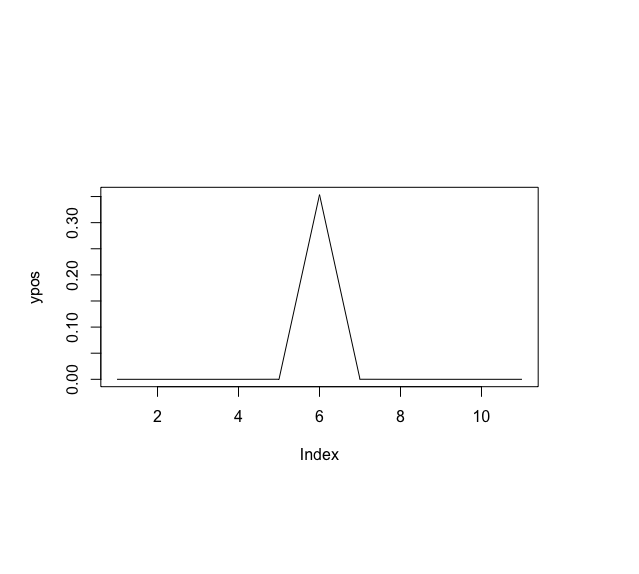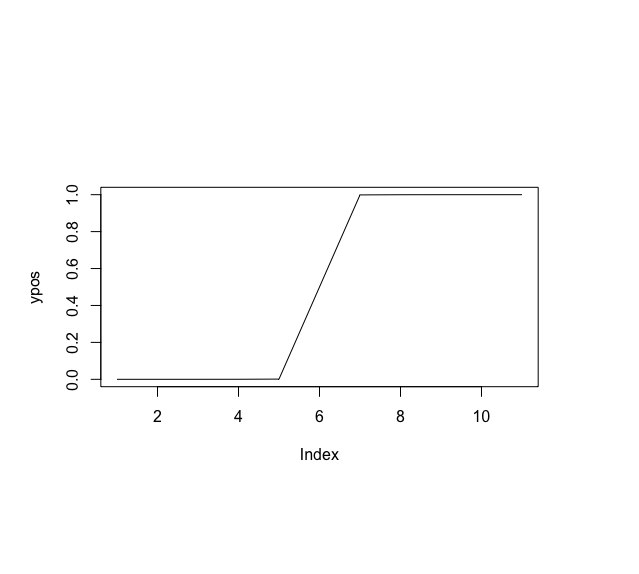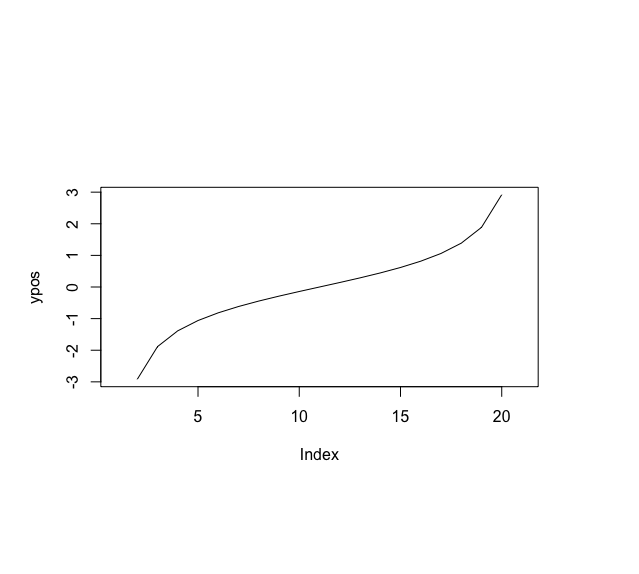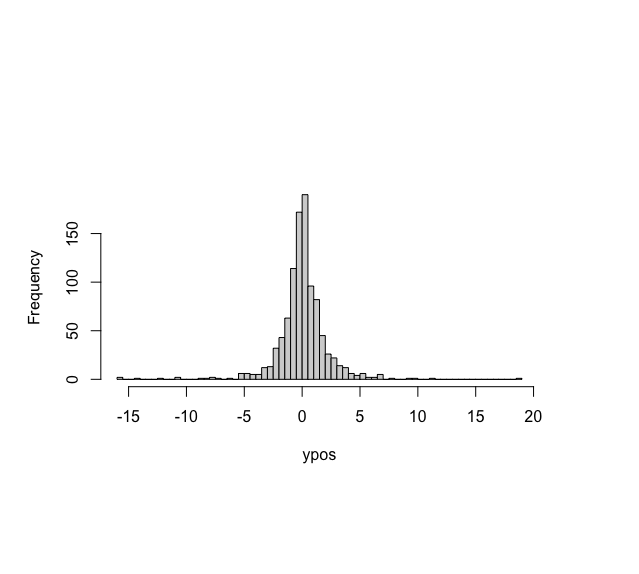Related Articles

# Plot t Distribution in R

• Last Updated : 27 Jul, 2021

The t-distribution, also known as the Student’s t-distribution is a type of probability distribution that is used to perform sampling of a normally distributed distribution where the sample size is small and the standard deviation of the input distribution is unknown. The distribution normally forms a bell curve, that is, the distribution is normally distributed but with a lower peak and more observations near the tail.

The t-distribution has only one associated parameter, called the degrees of freedom (df). The shape of a particular t-distribution curve relies on the number of degrees of freedom (df) chosen which is equivalent to the given sample size minus one, that is,

`df=n−1`

A vector of coordinates can be generated using the seq() method in R, which is used to generate an incremental sequence of integers to provide a distribution sequence for the given t-distribution. The corresponding y coordinates can be constructed using the various variants of the t-distribution function which are detailed below. These are then plotted using the plot() method in R programming language.

### dt() method

The dt() method in R is used to compute probability density analysis of the t-distribution with a specified degree of freedom.

Syntax:

dt(x, df )

Parameter :

• x – vector of quantiles
• df – degrees of freedom

Example:

## R

 `# generating x coordinates``xpos <- ``seq``(- 100, 100, by = 20) ` `print ``(``"X coordinates"``)``print ``(xpos)` `# generating y coordinates using dt() method``# degreesoffreedom``degree <- 2``ypos <- ``dt``(xpos, df = degree)` `print ``(``"Y coordinates"``)``print ``(ypos)` `# plotting t distribution``plot ``(ypos , type = ``"l"``)`

Output

 “X coordinates”

 -100  -80  -60  -40  -20    0   20   40   60   80  100

 “Y coordinates”

 9.997001e-07 1.952210e-06 4.625774e-06 1.559575e-05 1.240683e-04   3.535534e-01 1.240683e-04 1.559575e-05 4.625774e-06 1.952210e-06

 9.997001e-07### pt() method

The pt() method in R is used to produce a distribution function for a given student T-distribution. It is used to produce a cumulative distribution function. This function returns the area under the t-curve for any given interval.

Syntax:

pt(q, df, lower.tail = TRUE)

Parameter :

• q – quantile vector
• df – degrees of freedom
• lower.tail – if TRUE (default), probabilities are P[X ≤ x], otherwise, P[X > x].

Example:

## R

 `# generating x coordinates``xpos <- ``seq``(- 100, 100, by = 20) ` `print ``(``"X coordinates"``)``print ``(xpos)` `# generating y coordinates using dt() method``# degreesoffreedom``degree <- 2` `ypos <- ``pt``(xpos, df = degree)` `print ``(``"Y coordinates"``)``print ``(ypos)` `# plotting t distribution``plot ``(ypos , type = ``"l"``)`

Output

 “X coordinates”

 -100  -80  -60  -40  -20    0   20   40   60   80  100

 “Y coordinates”

 4.999250e-05 7.810669e-05 1.388310e-04 3.122073e-04 1.245332e-03   5.000000e-01 9.987547e-01 9.996878e-01 9.998612e-01 9.999219e-01

 9.999500e-01### qt() method

The qt() method in R is used to compute a quantile function or inverse cumulative density function for the given t-distribution for a specified number of degrees of freedom. It is used to compute the nth percentile of the student’s t-distribution with a specified degree of freedom.

Syntax:

qt(p, df, lower.tail = TRUE)

Parameter :

• p – vector of probabilities
• df – degrees of freedom
• lower.tail – if TRUE (default), probabilities are P[X ≤ x], otherwise, P[X > x].

Example:

## R

 `# generating x coordinates``xpos <- ``seq``(0, 1, by = 0.05)` `# generating y coordinates using dt() method``# degreesoffreedom``degree <- 2``ypos <- ``qt``(xpos, df = degree)` `# plotting t distribution``plot ``(ypos , type = ``"l"``)`

Output### rt() method

The rt() method is used for random generation for the t distribution using a specified number of degrees of freedom. n number of random samples may be generated.

Syntax:

rt(n, df)

Parameter :

• n – number of observations
• df – degrees of freedom

Example:

## R

 `# using a random number``n <- 1000` `# degreesoffreedom``degree <- 2` `# generating y coordinates using rt() method``ypos <- ``rt``(n , df = degree)` `# plotting t distribution in the form of``# distribution``hist``(ypos,                                       ``     ``breaks = 100,``     ``main = ``""``)`

OutputMy Personal Notes arrow_drop_up